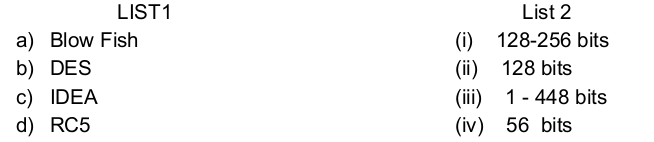GFG App
Open AppBrowser
Continue

# UGC-NET | UGC NET CS 2018 Dec – II | Question 36

Match the following secret key algorithm (List 1) with the corresponding key lengths (List 2) and choose the correct answer from the code given below.(A) (a)-(ii),(b)-(iii), (c)- (iv), (d)-(i)
(B) (a)-(iv),(b)-(iii), (c)- (ii), (d)-(i)
(C) (a)-(iii),(b)-(iv), (c)- (ii), (d)-(i)
(D) (a)-(iii),(b)-(iv), (c)- (i), (d)-(ii)

Explanation:

• Blowfish is a symmetric-key block cipher, it has a 64-bit block size and a variable key length from 32 bits up to 448 bits.
• DES we know works by taking a 64-bit value and a 56-bit key.
• IDEA (International Data Encryption Algorithm) operates on 64-bit blocks using a 128-bit key.
• Since at a time, RC5 uses 2 word blocks, the plain text block size can be 32, 64 or 128 bits.

Option (C) is correct.

Quiz of this Question

My Personal Notes arrow_drop_up